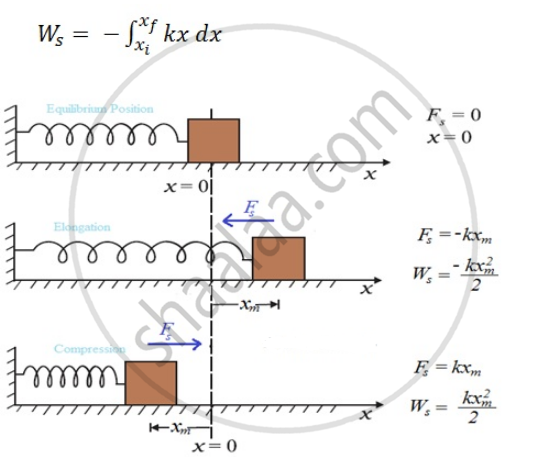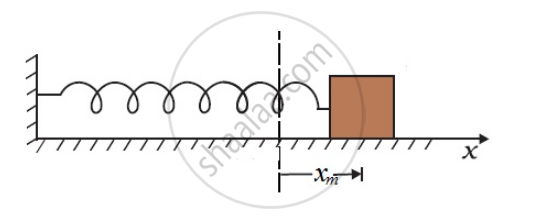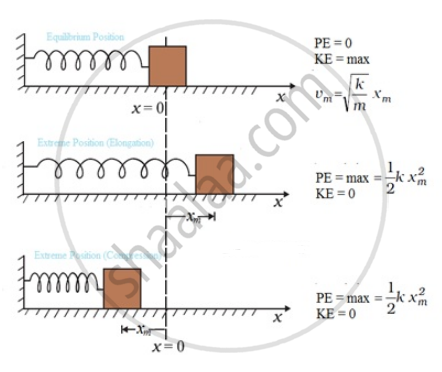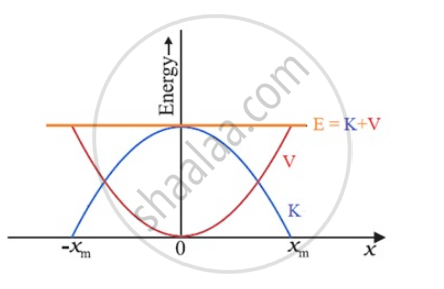# Potential Energy of a Spring

## Notes

#### Potential energy of spring

• The spring force is an example of a variable force, which is conservative.

• In an ideal spring, Fs = − kx , this force law for the spring is called Hooke’s law.

• The constant k is called the spring constant. Its unit is N m-1.

• The spring is said to be stiff if k is large and soft if k is small.Spring force is position dependent as first stated by Hooke,
Fs = − kx

• Work done by spring force only depends on the initial and final positions. Thus, the spring force is a conservative force.

• We define the potential energy V(x) of the spring to be zero when block and spring system is in the equilibrium position.

• If the extension is xm, the work done by the spring force is

W_s = ∫_0^(x_m) F_s  dx=-∫_0^(x_m) kx  dx
=-(kx_m^2)/2
The same is true when the spring is compressed with a displacement xc (< 0). The spring force does work Ws = -kx2/2 while the external force F does work + kxc^2/2. If the block is moved from an initial displacement xi to a final displacement xf, the work done by the spring force Ws is

W_s = -∫_(x_i)^(x_f) kx  dx = (kx_i^2)/2 - (kx_f^2)/2
Thus the work done by the spring force depends only on the end points. Specifically, if the block is pulled from xi and allowed to return to xi;
W_s = -∫_(x_i)^(x_f) kx  dx = (kx_i^2)/2 - (kx_f^2)/2 = 0
The work done by the spring force in a cyclic process is zero.

We have explicitly demonstrated that the spring force
(i) is position dependent only as first stated by Hooke, (Fs = − kx);
(ii) does work which only depends on the initial and final positions.
Thus, the spring force is a conservative force.

We define the potential energy V(x) of the spring to be zero when block and spring system is in the equilibrium position. For an extension (or compression) x the above analysis suggests that
V(x)=(kx^2)/2

If the block of mass m is extended to xm and released from rest, then its total mechanical energy at any arbitrary point x (where x lies between – xm and + xm) will be given by:
1/2 kx_m^2 = 1/2 kx^2 + 1/2 mv^2This suggests that the speed and the kinetic energy will be maximum at the equilibrium position, x = 0, i.e.,
1/2 mv_m^2 = 1/2 kx_m^2
where vm is the maximum speed.
Or v_m= sqrt(k/m)  x_m

Note that k/m has the dimensions of [T^(-2)] and our equation is dimensionally correct.The kinetic energy gets converted to potential energy and vice versa, however, the total mechanical energy remains constant. This is graphically depicted in FigExample: A car of mass M travelling with speed v, collides with a spring, having spring constant k. The car comes to rest (momentarily) when spring is compressed to a distance of x_m. Find x_m
Solution: Work done on car = K_f-K_i=0-(mv^2)/2 =-(mv^2)/2
Work done by spring, W_s= -∫_(x_i)^(x_f) kx  dx =-∫_(0)^(x_m) kx  dx = -(kx_m^2)/2
Now work done by spring equals work done on car => -(mv^2)/2 = - (kx_m^2)/2
x_m = sqrt (m/k)  v

If you would like to contribute notes or other learning material, please submit them using the button below.